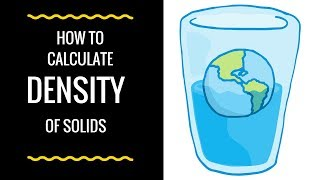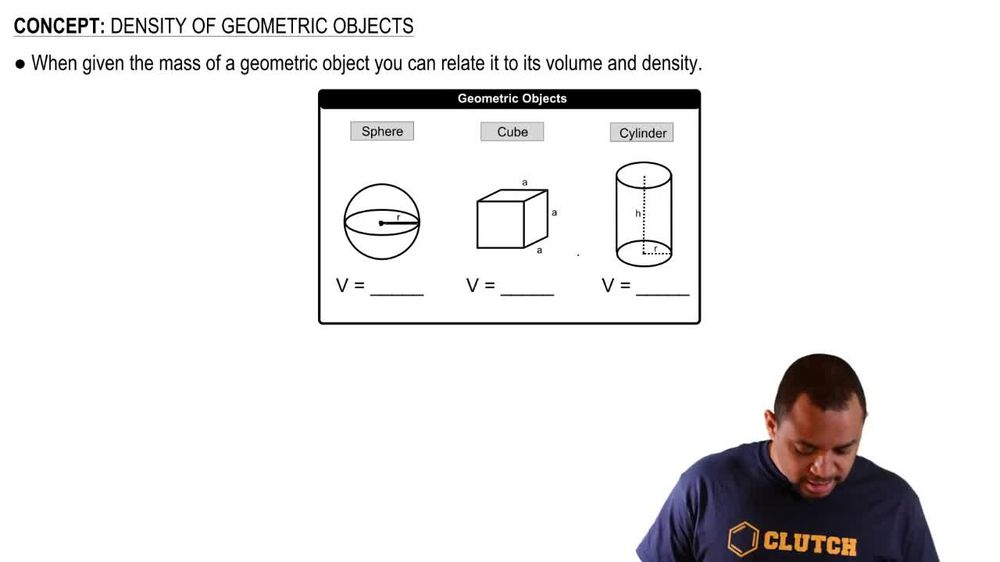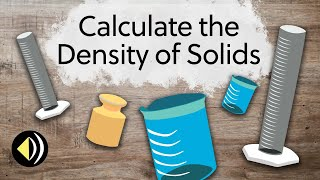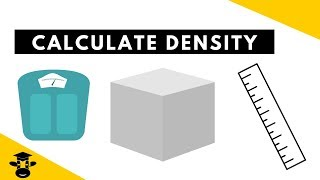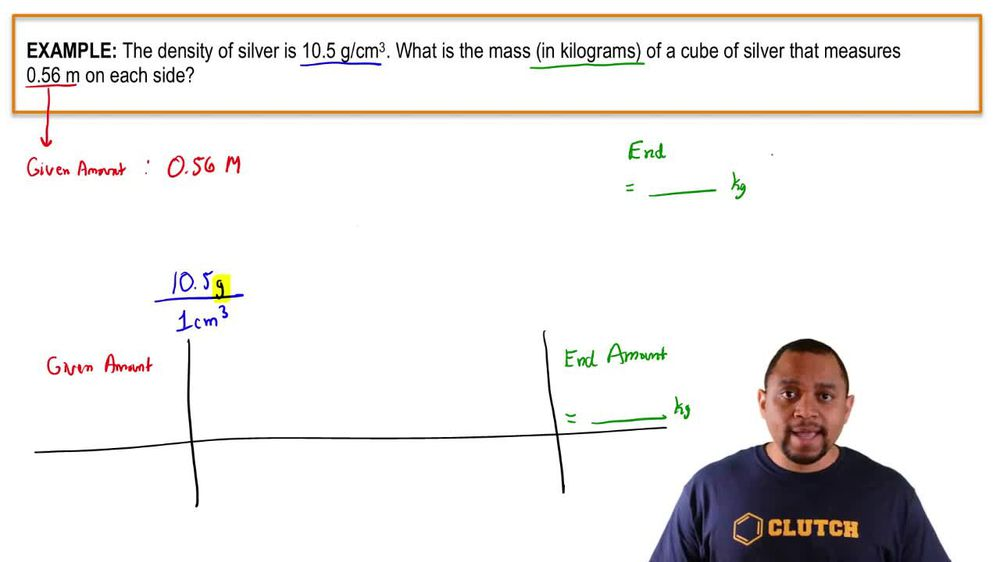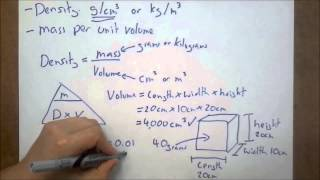Start typing, then use the up and down arrows to select an option from the list.
1. 1. Intro to General Chemistry2. Density of Geometric Objects
Problem

# The density of silver is 10.5 g/cm3. What is the mass (in kilo-grams) of a cube of silver that measures 0.62 m on each side?

Relevant Solution2m
Play a video:
Hi everyone here. We have a question telling us that a piece of a silver cube has an edge length of 6. cm. And our goal here is to calculate the mass of the Cube of the density of silver is 10.49 g per cm cubed. So we need to remember the formula density equals mass divided by volume, and the volume of a cube equals its side cubed, Which in this case is 6.50 cm. So we're going to keep that to get .625 cm cubed. And now we need to arrange our density equals mass over volume formula to get mass equals volume times density. And we know that our volume equals .625 centimeters cubed. And then we're going to multiply that by our density given to us in the problem which is 0.49 grams per centimeters cubed and our centimeters cubed are going to cancel out here. And that gives us 2880 g. And if we want to put that into scientific notation, we will take the decimal point 1 2, 3 places to the left. So 2.8, 8 Times 10 to the 3rd g. And that is our final answer. Thank you for watching. Bye